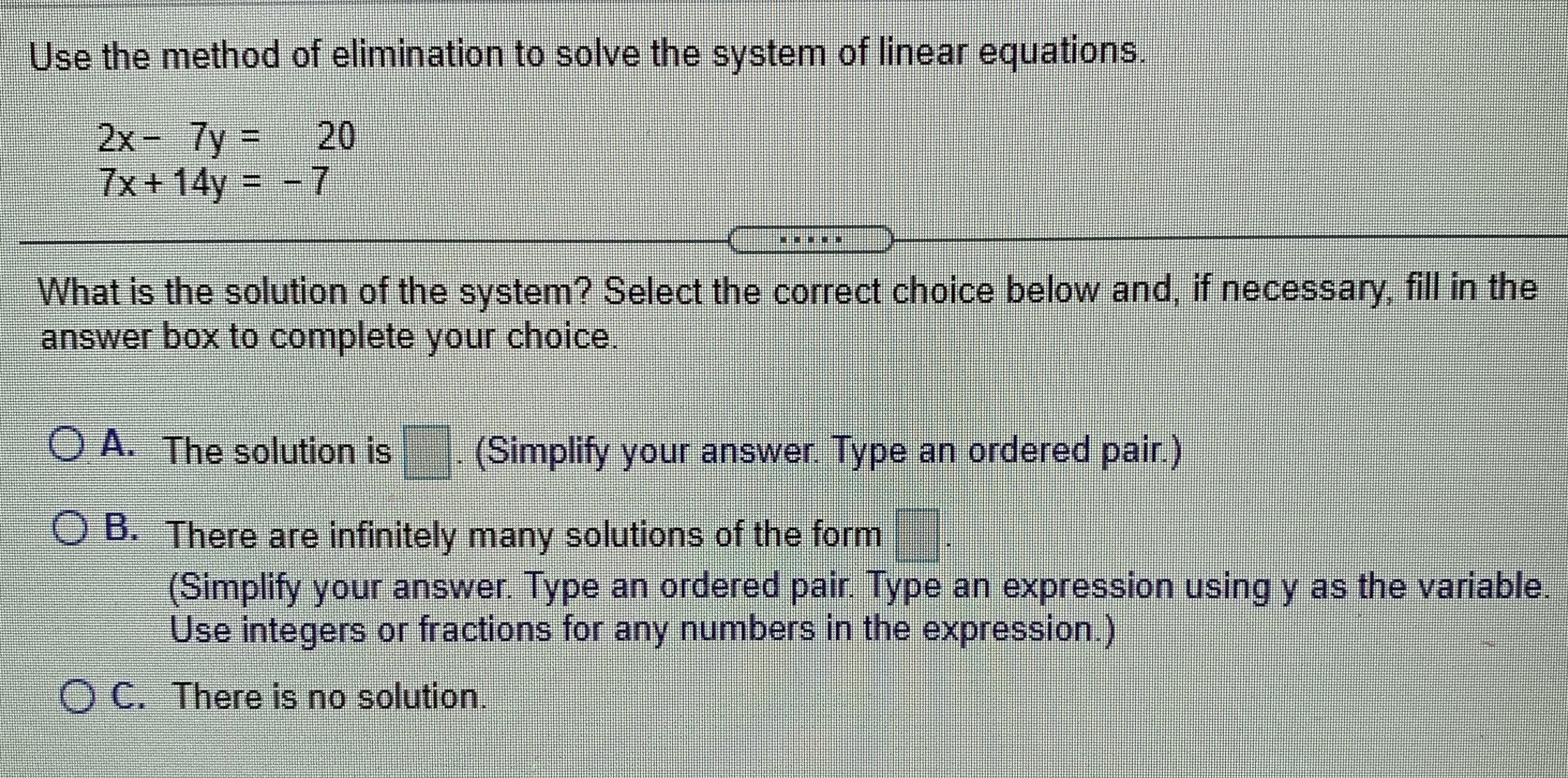### Still have math questions?

Algebra
QuestionUse the method of elimination to solve the system of linear equations.

$$2 x - 7 y = 20$$

$$7 x + 14 y = - 7$$

What is the solution of the system? Select the correct choice below and, if necessary, fill in the answer box to complete your choice.

A. The solution is $$\square$$ . (Simplify your answer. Type an ordered pair.)

B. There are infinitely many solutions of the form

(Simplify your answer. Type an ordered pair. Type an expression using y as the variable. Use integers or fractions for any numbers in the expression.)

C. There is no solution.